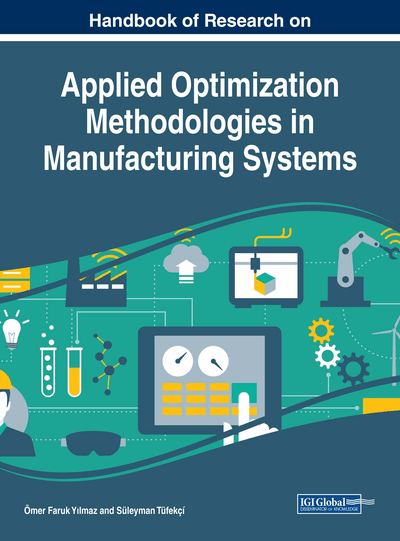# Heuristic Approaches in Clustering Problems

Onur Doğan (Istanbul Technical University, Turkey)
Copyright: © 2018 |Pages: 18
DOI: 10.4018/978-1-5225-2944-6.ch006
OnDemand PDF Download:
Available
\$37.50
No Current Special Offers

## Abstract

Clustering is an approach used in data mining to classify objects in parallel with similarities or separate according to dissimilarities. The aim of clustering is to decrease the amount of data by grouping similar data items together. There are different methods to cluster. One of the most popular techniques is K-means algorithm and widely used in literature to solve clustering problem is discussed. Although it is a simple and fast algorithm, there are two main drawbacks. One of them is that, in minimizing problems, solution may trap into local minimum point since objective function is not convex. Since the clustering is an NP-hard problem and to avoid converging to a local minimum point, several heuristic algorithms applied to clustering analysis. The heuristic approaches are a good way to reach solution in a short time. Five approaches are mentioned briefly in the chapter and given some directions for details. For an example, particle swarm optimization approach was used for clustering problem. In example, iris dataset including 3 clusters and 150 data was used.
Chapter Preview
Top

## Introduction

Researchers have studied for years to find optimal solutions to the problems (Reeves, 1995). The problem searching the optimum solution according to decision variable is called optimization problem. The main purpose of an optimization problem is to maximize or minimize a function which is called objective function. The objective function can sometimes be maximizing profit or minimizing total cost of transportation. “Mathematical models” are a bridge between the mathematic and real world (Meerschaert, 2013). If x refers to decision variables vector, the objective function of the problem depending on the decision variables is f(x). A mathematical model of a minimization problem can be shown as follows:

minimize(1) subject to(2)(3)

Most of the real world problems contain multiple objectives and contradictory criteria (Singh & Yadav, 2015). If a mathematical model consists of more than one objective function, it is called multi-objective programming. In this case, the objective function iswhere g(x), h(x) and l(x) show objective functions.

Optimization problems are classified two main categories, continuous and discrete optimization. While decision variables can take any value in continuous optimization problems, in discrete problems, they can take predefined values in solution space such as taking integer values (Cura T., 2008).

In real life problems, finding the best solution is usually take so much time due to infinite solution space. It is expected to find a result near to the best solution in an acceptable time. Using some rules and some solutions instead of all of them, heuristic algorithms reach near to an optimum solution. It does not guarantee to find the best solution. They reduce the time consumption and give the flexibility (Bassett, 2000; Yavuz, Inan, & Fığlalı, 2008).

Although, in operation research problem, searching a solution to a problem, the first step is to create a model, in this chapter, it is considered independent from mathematical model.

Due to clustering problem is an NP-hard problem (Aloise, Deshpande, & Hansen, 2009; Dasgupta, 2007; Drineas, Frieze, Kannan, Vempala, & Vinay, 2004), heuristic algorithms can be used to solve it. Clustering analysis is a type of data mining methods. The goal of the clustering is to create groups according to similarities among the individuals and dissimilarities among the groups. It uses distances to calculate similarities or dissimilarities. There are different ways to calculate it, Euclidean, Pearson, Manhattan, Minkowski etc. Euclidean distance between two objects is commonly used in literature.

## Complete Chapter List

Search this Book:
Reset